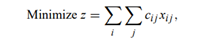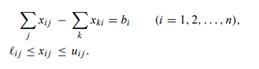# The general minimum-cost flow problem is given as follows

The general minimum-cost flow problem is given as follows:Subject to:a) Assuming that the lower bounds on the variables are all finite, this is, `i j > −∞, show that any problem of this form can be converted to a transportation problem with lower bounds on the variables of zero and nonnegative, or infinite, upper bounds. (Hint. Refer to Exercise 22.)

b) Comment on the efficiency of solving minimum-cost flow problems by a bounded-variables transportation method, versus the simplex method for general networks